# MHKiT WEC-Sim Example

This example loads simulated data from a WEC-Sim run for a two-body point absorber (Reference Model 3) and demonstrates the application of the MHKiT wave module to interact with the simulated data. The analysis is broken down into three parts: load WEC-Sim data, view and interact with the WEC-Sim data , and apply the MHKiT wave module.

2. WEC-Sim Simulated Data

• Wave Class Data

• Body Class Data

• PTO Class Data

• Constraint Class Data

• Mooring Class Data

3. Apply MHKiT Wave Module

Start by importing MHKiT and the necessary python packages (e.g.scipy.io and matplotlib.pyplot).

:

from  mhkit import wave
import scipy.io as sio
import matplotlib.pyplot as plt


## 1. Load WEC-Sim Simulated Data

WEC-Sim saves output data as a MATLAB output object, generated by WEC-Sim’s Response Class. The WEC-Sim output object must be converted to a structure for use in Python.

Here we will load the WEC-Sim RM3 data run with a mooring matrix.

:

# Relative location and filename of simulated WEC-Sim data (run with mooring)
filename = './data/wave/RM3MooringMatrix_matlabWorkspace_structure.mat'

# Load data using the wecsim.read_output function which returns a dictionary of dataFrames

moorDyn class not used
ptosim class not used
cable class not used


NOTE: The mhkit.wave.io.wecsim.read_output function prints a message letting the user know which WEC-Sim classes were not used. This WEC-Sim example data for the RM3 was not run with MoorDyn, PTO-Sim or a cable.

NOTE: Conversion of the WEC-Sim object to a struct must be done in MATLAB and can be achieved using the command: output = struct(output);.

## 2. WEC-Sim Simulated Data

This section will investigate the WEC-Sim RM3 data loaded using MHKiT. In the previous section mhkit.wave.io.wecsim.read_output returned a dictonary of DataFrames in a format similar to the WEC-Sim output object. To see what data is available we can call the keys method on the wecsim_data dictionary:

:

# View the available WEC-Sim data, a dataFrame for each WEC-Sim Class
wecsim_data.keys()

:

dict_keys(['wave', 'bodies', 'ptos', 'constraints', 'mooring', 'moorDyn', 'ptosim', 'cables'])


We can see above that there are eight returned keys. As noted from the mhkit.wave.io.wecsim.read_output function the moorDyn, ptosim and cables keys will be empty as the function found the data missing from the output. The following sections will work through each of the 5 other WEC-Sim classes.

### Wave Class Data

Data from WEC-Sim’s Wave Class includes information about the wave input, including the the wave type, and wave elevation as a function of time. Below we will wave the wave DataFrame as wave and then look at the wave type and the top (head) of the DataFrame.

:

# Store WEC-Sim output from the Wave Class to a new dataFrame, called wave_data
wave_data = wecsim_data['wave']

# Display the wave type from the WEC-Sim Wave Class
wave_type = wave_data.name
print("WEC-Sim wave type:", wave_type)

# View the WEC-Sim output dataFrame for the Wave Class
wave_data

WEC-Sim wave type: elevationImport

:

elevation
time
0.00 -0.000000e+00
0.01 -2.766568e-07
0.02 -1.106006e-06
0.03 -2.486738e-06
0.04 -4.416811e-06
... ...
399.96 -3.480172e-01
399.97 -3.429291e-01
399.98 -3.378586e-01
399.99 -3.326988e-01
400.00 -3.274562e-01

40001 rows × 1 columns

The WEC-Sim wave type refers to the type of wave used to run the WEC-Sim simulation. Data from this WEC-Sim run was generated used ‘etaImport’, meaning a user-defined wave surface elevation was defined. For more information about the wave types used by WEC-Sim, refer to the Wave Class documentation.

#### Plot the Wave Elevation Data

We can use the pandas DataFrame plot method to quickly view the Data. The plot method will set the index (time) on the x-axis and any columns (in this case only wave elevation) on the y-axis.

:

# Plot WEC-Sim output from the Wave Class
wave_data.plot()
plt.xlabel("Time [s]")
plt.ylabel("Wave Surface Elevation [m]")

:

Text(0, 0.5, 'Wave Surface Elevation [m]')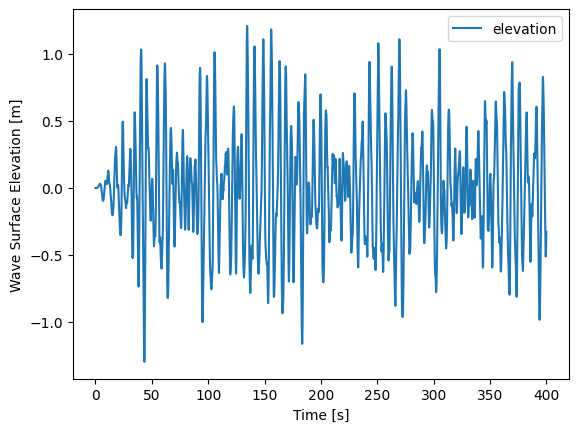### Body Class Data

Data from WEC-Sim’s Body Class includes information about each body, including the body’s position, velocity, acceleration, forces acting on the body, and the body’s name. For the RM3 example there will be 2 boides a float and a spar.

:

# Store WEC-Sim output from the Body Class to a new dictionary of dataFrames, i.e. 'bodies'.
bodies = wecsim_data['bodies']

# Data fron each body is stored as its own dataFrame, i.e. 'body1' and 'body2'.
bodies.keys()

:

dict_keys(['body1', 'body2'])


#### Body Class Data for Body 1

Let us determine which body ‘body1’ is by requesting the body’s name. In this this case the body name is ‘float’.

:

# Store Body Class dataFrame for Body 1 as body1.
body1 = bodies['body1']

# Display the name of Body 1 from the WEC-Sim Body Class
print("Name of Body 1:", body1.name)

Name of Body 1: float


For a given body WEC-Sim returns simulated parameters (the number of simulated parameters varies by the options choosen) for each of the 6 degrees of freedom (DOF). We can view the unique simulated parameters by filtering the columns by a single DOF. For this example we look at surge data (DOF 1).

:

# Print a list of Body 1 columns that end with 'dof1'
[col for col in body1 if col.endswith('dof1')]

:

['position_dof1',
'velocity_dof1',
'acceleration_dof1',
'forceTotal_dof1',
'forceExcitation_dof1',
'forceRestoring_dof1',
'forceMorisonAndViscous_dof1',
'forceLinearDamping_dof1']

##### Plot heave position data for Body 1

The RM3 device creates power in the heave direction (DOF 3). Let us consider the float’s (body 1) position as a function of time by plotting postion_dof3.

:

# Use Pandas to plot Body 1 position in heave (DOF 3)
body1.position_dof3.plot()
plt.xlabel("Time [s]")
plt.ylabel("Heave Position [m]")
plt.title('Body 1')

# Use Pandas to calculate the maximum and minimum heave position of Body 1
print("Body 1 max heave position =", body1.position_dof3.max(),"[m]")
print("Body 1 min heave position =", body1.position_dof3.min(),"[m]")

Body 1 max heave position = -0.09745294999054366 [m]
Body 1 min heave position = -1.3264489287377081 [m]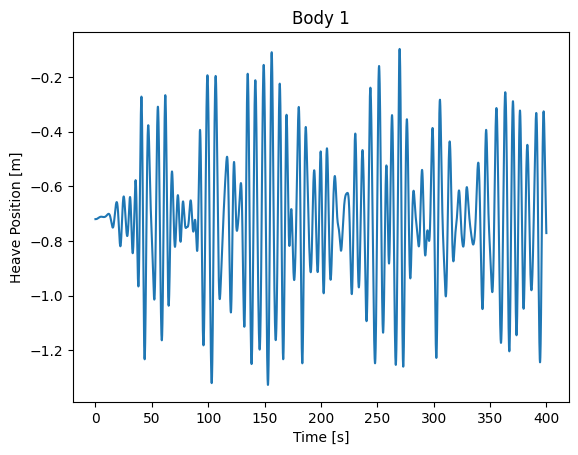##### Plot Body 1 position data for all DOFs

As an example we could plot multiple postion DOFs by calling a plot on only the position columns. To do that we would first create a list of stings (filter_col) which include the string 'position'. Then slicing the DataFrame on that list of strings and calling plot will plot the position over time of all 6 degrees of freedom for body1.

:

# Create a list of Body 1 data columns that start with 'position'
filter_col = [col for col in body1 if col.startswith('position')]

# Plot filtered 'position' data for Body 1
body1[filter_col].plot()
plt.xlabel('Time [s]')
plt.title('Body 1')
plt.legend(loc='center left', bbox_to_anchor=(1, 0.5))

:

<matplotlib.legend.Legend at 0x204dea0fdf0>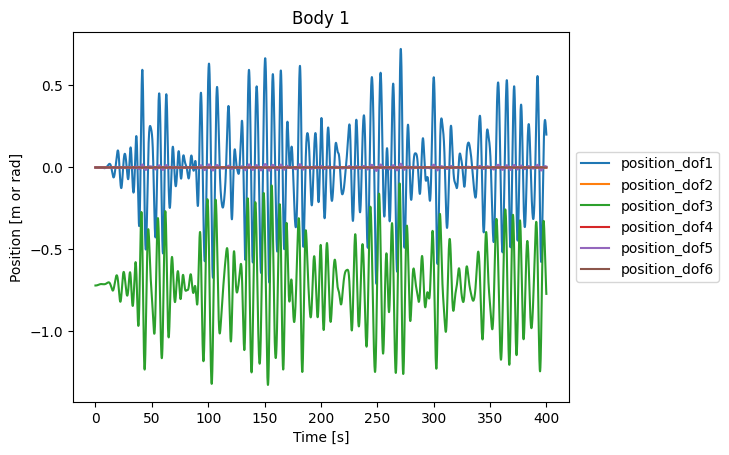#### Body Class Data for Body 2

We can look at the name of body 2 to see that is the ‘sapr’ of RM3. Looking at the top of the body2 DataFrame we see the same available data as body1.

:

# Store Body Class dataFrame for Body 2 as body2
body2 = bodies['body2']

# Display the name of Body 2 from the WEC-Sim Body Class
print("Name of Body 2:", body2.name)

# View the Body Class dataFrame for Body 2

Name of Body 2: spar

:

time
0.00 0.000000e+00 0.000000e+00 3.755268e-11 0.002335 0.000000 0.0 -0.002335 0.0 0 0 ... 0.0 0.0 0.0 -0.000032 0.000000e+00 0.0 0.000032 0.0 0 0
0.01 1.591394e-14 3.896569e-12 4.658216e-10 0.003717 0.000114 0.0 -0.003603 0.0 0 0 ... 0.0 0.0 0.0 -0.000049 3.542912e-11 0.0 0.000049 0.0 0 0
0.02 1.060269e-13 1.600503e-11 1.593218e-09 0.005343 0.000471 0.0 -0.004872 0.0 0 0 ... 0.0 0.0 0.0 -0.000068 1.419347e-10 0.0 0.000068 0.0 0 0
0.03 3.850573e-13 4.276107e-11 3.369237e-09 0.006388 0.001097 0.0 -0.005291 0.0 0 0 ... 0.0 0.0 0.0 -0.000079 3.198299e-10 0.0 0.000079 0.0 0 0
0.04 1.024419e-12 8.867535e-11 5.507643e-09 0.006671 0.002016 0.0 -0.004656 0.0 0 0 ... 0.0 0.0 0.0 -0.000081 5.694090e-10 0.0 0.000081 0.0 0 0

5 rows × 60 columns

### PTO Class Data

Data from WEC-Sim’s PTO Class includes information about each PTO, including the PTO’s position, velocity, acceleration, forces acting on the PTO, and the PTO’s name. In this case there is only 1 PTO.

:

# Store WEC-Sim output from the PTO Class to a DataFrame, called ptos
ptos = wecsim_data['ptos']

# Display the name of the PTO from the WEC-Sim PTO Class
print("Name of PTO:", ptos.name)

# Print a list of available columns that end with 'dof1'
[col for col in ptos if col.endswith('dof1')]

Name of PTO: PTO1

:

['position_dof1',
'velocity_dof1',
'acceleration_dof1',
'forceTotal_dof1',
'forceActuation_dof1',
'forceConstraint_dof1',
'forceInternalMechanics_dof1',
'powerInternalMechanics_dof1']

:

# Use Pandas to plot pto internal power in heave (DOF 3)
# NOTE: WEC-Sim requires a negative sign to convert internal power to generated power
(-1*ptos.powerInternalMechanics_dof3/1000).plot()
plt.xlabel("Time [s]")
plt.ylabel("Power Generated [kW]")
plt.title('PTO')

:

Text(0.5, 1.0, 'PTO')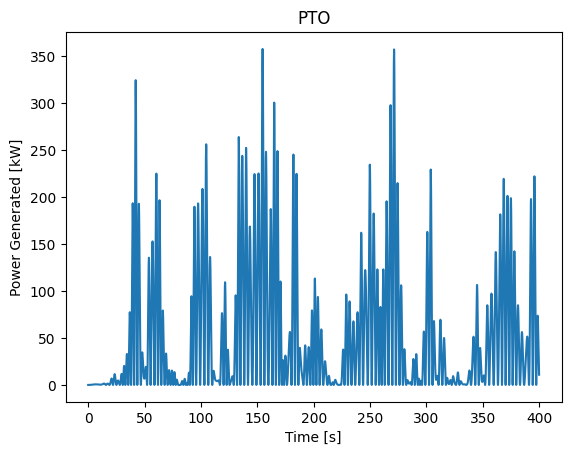### Constraint Class Data

Data from WEC-Sim’s Constraint Class includes information about each constraint, including the constraint’s position, velocity, acceleration, forces acting on the constraint, and the constraint’s name.

:

# Store WEC-Sim output from the Constraint Class to a new dataFrame, called constraints
constraints = wecsim_data['constraints']

# Display the name of the Constraint from the WEC-Sim Constraint Class
print("Name of Constraint:", constraints.name)

# View the Constraint Class dataFrame

Name of Constraint: Constraint1

:

position_dof1 velocity_dof1 acceleration_dof1 forceConstraint_dof1 position_dof2 velocity_dof2 acceleration_dof2 forceConstraint_dof2 position_dof3 velocity_dof3 ... acceleration_dof4 forceConstraint_dof4 position_dof5 velocity_dof5 acceleration_dof5 forceConstraint_dof5 position_dof6 velocity_dof6 acceleration_dof6 forceConstraint_dof6
time
0.00 0.000000e+00 0.000000e+00 -2.382256e-10 0.0 0.0 0.0 0.0 1.615587e-27 0.000000e+00 0.000000 ... 0.0 -3.636025 -0.000000e+00 -0.000000e+00 -1.282689e-11 -0.0 0.0 0.0 0.0 -7.703720e-34
0.01 -7.759428e-14 -1.900049e-11 -2.327210e-09 0.0 0.0 0.0 0.0 1.198363e-03 1.828372e-07 0.000039 ... 0.0 -3.325180 -4.349220e-15 -1.064980e-12 -1.299084e-10 -0.0 0.0 0.0 0.0 3.168962e-05
0.02 -4.942732e-13 -7.203815e-11 -6.948927e-09 0.0 0.0 0.0 0.0 2.493818e-03 8.204028e-07 0.000091 ... 0.0 -2.984545 -2.792094e-14 -4.095032e-12 -3.973091e-10 -0.0 0.0 0.0 0.0 6.653122e-05
0.03 -1.677273e-12 -1.739122e-10 -1.255916e-08 0.0 0.0 0.0 0.0 3.245134e-03 2.036966e-06 0.000154 ... 0.0 -2.781700 -9.592234e-14 -1.007783e-11 -7.408555e-10 -0.0 0.0 0.0 0.0 8.740994e-05
0.04 -4.117573e-12 -3.216348e-10 -1.705139e-08 0.0 0.0 0.0 0.0 3.345214e-03 3.906281e-06 0.000220 ... 0.0 -2.746425 -2.391624e-13 -1.908419e-11 -1.049258e-09 -0.0 0.0 0.0 0.0 9.123989e-05

5 rows × 24 columns

### Mooring Class Data

Data from WEC-Sim’s Mooring Class includes relevant information about the mooring, including the mooring’s position, velocity, mooring force, and the mooring’s name.

:

# Store WEC-Sim output from the Mooring Class to a new dataFrame, called mooring
mooring = wecsim_data['mooring']

# View the PTO Class dataFrame

:

position_dof1 velocity_dof1 forceMooring_dof1 position_dof2 velocity_dof2 forceMooring_dof2 position_dof3 velocity_dof3 forceMooring_dof3 position_dof4 velocity_dof4 forceMooring_dof4 position_dof5 velocity_dof5 forceMooring_dof5 position_dof6 velocity_dof6 forceMooring_dof6
time
0.00 0.000000e+00 0.000000e+00 0.000000e+00 0.0 0.0 0.0 0.000000e+00 0.000000 0.0 0.0 0.0 0.0 -0.000000e+00 -0.000000e+00 0.0 0.0 0.0 0.0
0.01 -7.759428e-14 -1.900049e-11 7.759428e-09 0.0 0.0 0.0 1.828372e-07 0.000039 0.0 0.0 0.0 0.0 -4.349220e-15 -1.064980e-12 0.0 0.0 0.0 0.0
0.02 -4.942732e-13 -7.203815e-11 4.942732e-08 0.0 0.0 0.0 8.204028e-07 0.000091 0.0 0.0 0.0 0.0 -2.792094e-14 -4.095032e-12 0.0 0.0 0.0 0.0
0.03 -1.677273e-12 -1.739122e-10 1.677273e-07 0.0 0.0 0.0 2.036966e-06 0.000154 0.0 0.0 0.0 0.0 -9.592234e-14 -1.007783e-11 0.0 0.0 0.0 0.0
0.04 -4.117573e-12 -3.216348e-10 4.117573e-07 0.0 0.0 0.0 3.906281e-06 0.000220 0.0 0.0 0.0 0.0 -2.391624e-13 -1.908419e-11 0.0 0.0 0.0 0.0

## 3. Apply MHKiT Wave Module

In the example below we will calculate a spectrum from the wecsim wave elevation timeseries data using the MHKiT elevation_spectrum function.

:

# Use the MHKiT Wave Module to calculate the wave spectrum from the WEC-Sim Wave Class Data
sample_rate=60
nnft=1000        # Number of bins in the Fast Fourier Transform
ws_spectrum = wave.resource.elevation_spectrum(wave_data, sample_rate, nnft)

# Plot calculated wave spectrum
spect_plot = wave.graphics.plot_spectrum(ws_spectrum)
spect_plot = spect_plot.set_xlim([0, 4])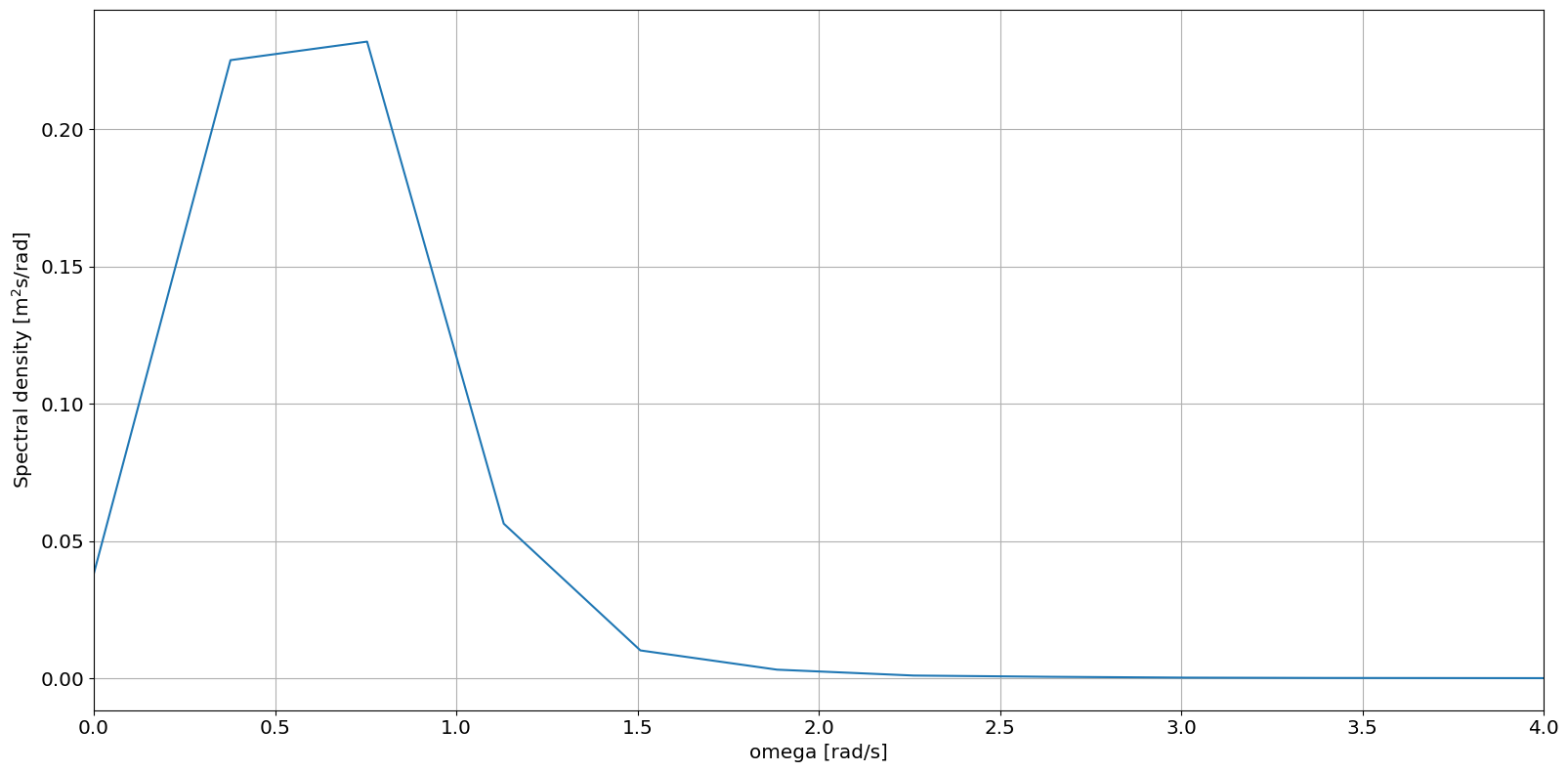:

# Calculate Peak Wave Period (Tp) and Significant Wave Height (Hm0)
Tp = wave.resource.peak_period(ws_spectrum)
Hm0 = wave.resource.significant_wave_height(ws_spectrum)

# Display calculated Peak Wave Period (Tp) and Significant Wave Height (Hm0)
display(Tp,Hm0)

Tp
elevation 8.333333
Hm0
elevation 1.785851